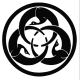### Some infinite series

Problem:

Find the sum of the series:
\begin{align} & \sum_{n=0}^\infty \frac{n^4}{n!} \\ & \sum_{n=0}^\infty \frac{(n+1)^2}{n!}\\ & \sum_{n=0}^\infty \frac{(n+1)^3}{n!}\; . \end{align}
Sono sempre stato, e mi ritengo ancora un dilettante. Cioè una persona che si diletta, che cerca sempre di provare piacere e di regalare il piacere agli altri, che scopre ogni volta quello che fa come se fosse la prima volta. (Freak Antoni)gugo82
Moderatore globaleMessaggio: 22627 di 24357
Iscritto il: 12/10/2007, 23:58
Località: Napoli

### Re: Some infinite series

gugo82 ha scritto:Problem:

Find the sum of the series:
\begin{align} & \sum_{n=0}^\infty \frac{n^4}{n!} \\ & \sum_{n=0}^\infty \frac{(n+1)^2}{n!}\\ & \sum_{n=0}^\infty \frac{(n+1)^3}{n!}\; . \end{align}

Testo nascosto, fai click qui per vederlo
(1) We can rewrite:
$\sum_{n=0}^\infty \frac{n^4}{n!} = \sum_{n=1}^\infty \frac{n^3}{(n-1)!} = \sum_{n=0}^\infty \frac{(n+1)^3}{n!}$
hence the sum of (1) equals the sum of (3), which will be evaluated in a while.

(2) We have:
$\sum_{n=0}^\infty \frac{(n+1)^2}{n!} = \sum_{n=0}^\infty \frac{n^2 + 2n +1}{n!} = \sum_{n=0}^\infty \frac{n(n-1) +3n + 1}{n!} = \sum_{n=2}^\infty \frac{1}{(n-2)!} + 3 \sum_{n=1}^\infty \frac{1}{(n-1)!} + \sum_{n=0}^\infty \frac{1}{n!} = e + 3e + e = 5e\;.$

(3) We find:
$\sum_{n=0}^\infty \frac{(n+1)^3}{n!} = \sum_{n=0}^\infty \frac{n^3 + 3n^2 + 3n +1}{n!} = \sum_{n=0}^\infty \frac{n(n-1)(n-2) + 6n(n-1) + 7n +1}{n!} = \sum_{n=3}^\infty \frac{1}{(n-3)!} + 6 \sum_{n=2}^\infty \frac{1}{(n-2)!} + 7 \sum_{n=1}^\infty \frac{1}{(n-1)!} + \sum_{n=0}^\infty \frac{1}{n!} = e + 6e + 7e + e = 15e\; .$

***

Is it possibile to write down an explicit formula for $sum_(n=0)^oo (n+k)^2/(n!)$ and $sum_(n=0)^oo (n+k)^3/(n!)$ (with $k in NN$)?
And what about a formula for $sum_(n=0)^oo (n+1)^h/(n!)$ (with $h in NN$)?
Sono sempre stato, e mi ritengo ancora un dilettante. Cioè una persona che si diletta, che cerca sempre di provare piacere e di regalare il piacere agli altri, che scopre ogni volta quello che fa come se fosse la prima volta. (Freak Antoni)gugo82
Moderatore globaleMessaggio: 22815 di 24357
Iscritto il: 12/10/2007, 23:58
Località: Napoli

### Re: Some infinite series

Testo nascosto, fai click qui per vederlo
Since $$(1) = (3)$$ (after a simplification and a change of variable), we focus on $$(2)$$ and $$(3)$$.

$$(2) .$$ We have that $\sum_{n = 0}^\infty \frac{(n+1)^2}{n!} = \sum_{n = 0}^\infty \frac{n^2}{n!} + \frac{2n}{n!} + \frac{1}{n!}.$Since everything here is absolutely convergent we can harmlessly permute the summation order: $\sum_{n = 0}^\infty \frac{n}{n!} = \sum_{n = 1}^\infty \frac{1}{(n-1)!} = e$ and $\sum_{n = 0}^\infty \frac{n^2}{n!} = \sum_{n = 1}^\infty \frac{n}{(n-1)!} = \sum_{n=0}^\infty \frac{n+1}{n!} = 2e.$ In conclusion the sum of $$(2)$$ equals $$5 e$$.

$$(3).$$ With similar computations it turns out that the sum of $$(3)$$ equals $$15 e$$.

I would like to suggest a problem, too:

Using $$\sum_{n=1}^\infty \frac{1}{n^2}= \frac{\pi^2}{6}$$, compute $\sum_{n=1}^\infty \frac{1}{(n+2)^2} \left( \frac{1}{1 \cdot 2} + \frac{1}{2 \cdot 3} + \dots + \frac{1}{n \cdot (n+1)}\right).$
consumami
distruggimi
è un po' che non mi annoio
obnoxious
Junior MemberMessaggio: 141 di 208
Iscritto il: 22/03/2019, 11:45

Torna a The English Corner

### Chi c’è in linea

Visitano il forum: Nessuno e 4 ospiti